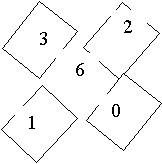#### You may also like### Prompt Cards

These two group activities use mathematical reasoning - one is numerical, one geometric.### Consecutive Numbers

An investigation involving adding and subtracting sets of consecutive numbers. Lots to find out, lots to explore.### Exploring Wild & Wonderful Number Patterns

EWWNP means Exploring Wild and Wonderful Number Patterns Created by Yourself! Investigate what happens if we create number patterns using some simple rules.

# X Is 5 Squares

##### Age 7 to 11Challenge Level

A solution came from Tom of Lexden Primary School in Colchester, Essex. Tom explains how he set about solving the problem.

"I worked out what possibilities there were for multiplying the top two numbers together to equal the middle number. The two possibilities were 6 and 8 for the middle number.

I then worked out the other outside numbers so that when I added them altogether they would make 6 or 8. 8 didn't work."

So what did work? Tom knew, so did Ben and Thomas both pupils at Montgomery Church in Wales School, Powys.

They explain: "The middle number has to be 6 because that is the lowest number that is the one that four of the other lower numbers can total. That leaves 1, 2, 3 and 0 to be used around the outside. We multiplied pairs of these numbers together until we found one pair that made 6. These numbers were 2 and 3."

As Tom said, "The solution won't mirror image top to bottom because the top two numbers have to multiply to make the middle number."

Ben and Thomas agreed. They completed the task this way: "1 and 0 were then used to make the diagonal lines total the same amount."Emma and Francine found the same solution. They showed it the same way as Tom.

Well done to each of you. Are there any other series of consecutive numbers that will allow you to make a similar puzzle? Abigail who goes to Histon and Impington Infants School sent us in a very detailed solution to this problem. She wrote:
We named the squares with letters and then we wrote down the three rules in letters:
A B
C
D E

1. AxB=C 2. D+E=C 3. B+C+D=A+C+E I then made a guess, that if A=6 and if B=4, C must be 24, but this is too big, so B must be smaller. I tried B=2 which is still too big, then B=1, but then C=6, but this is not different to A, so A cannot be 6.

I guessed that A might be 1 and B=2, but then C = 2 too. I saw that A can't be 1 because then B and C would be the same.

I guessed that A might be 5, and tried B=2, but then C is too big, but B can't be 1 because A would be the same as C so I tried another value for A.

I guessed that A might be 3, and B =2 then C=6 which is possible. I tried other B values.. Not 1, not 3, maybe 4, but that is too big.

Then I guessed A might be 2. If A = 2 and B=3, C=6 is possible. If A= 2 and B=4, C=8 is possible. B can't be 5 because C would have to be 10. B can't be bigger than 5 when A is 2.

I then wrote down what I had found so far.
A = 6 is not possible
A = 1 is not possible
A = 5 is not possible
A = 3 is possible
A = 2 is possible

We could try A = 4, but we don't need to try bigger than 6, because then C would be too big.

So if A = 4. B could be 2 and C could be 8. But if B is 3, C is too big.

With all of the possible answers, C is either 6 or 8.
Then I looked at D + E = C. If C = 6

I wrote a table:
D E
1 5
2 4
3 3
4 2
5 1
0 6
6 0

D or E can't be 2 because 2 is already used in A or B. They can't be 3 because they need to be different. They can't be 6 because that is C.
If C = 8, D and E could be...
D E
0 8 but C=8
1 7
2 6 but A or B = 2
5 3
4 4 but they can't be the same 3 5 6 2 but A or B = 2
7 1
8 0 but C =8

Then Mummy helped me write a big table with all the possible answers, and I worked out the sums for rule 3:
A B C D E B+C+D A+C+E
4 2 8 1 7 11 19
4 2 8 5 3 15 15 OK
4 2 8 3 5 13 17
4 2 8 7 1 17 13
2 4 8 1 7 13 17
2 4 8 5 3 17 13
2 4 8 3 5 15 15 OK
2 4 8 7 1 19 11
2 3 6 1 5 10 13
2 3 6 5 1 14 9
3 2 6 1 5 9 14
3 2 6 5 1 13 10